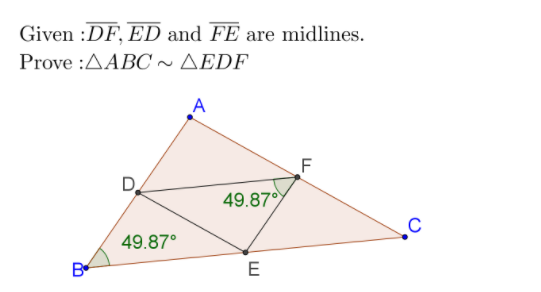# Geo-2017-Construction-Similar Triangles

## AA Similarity Theorem

If two triangles have exactly two pairs of corresponding angles that are congruent, then the triangles are similar.
1. Draw any triangle using the polygon tool at the top of the screen.
2. Measure the lengths of each sides.
3. Use the angle tool to measure the angles at each vertex.
4. Construct the midpoint of the each side.
5. Connect the midpoints to make a triangle.
6. Measure the perimeter of each triangle using the following command: `Perimeter[poly1] `

## SAS Similarity ProofSAS Similarity Theorem: If two triangle have two pairs of proportional sides and the included angles are congruent then the triangles are similar. ﻿

Prove that ABC and the triangle formed by its midlines [segments connecting the midpoints of each side] are similar.

## Similar Triangles: Scale Ratio

1. Draw the triangle formed by the midpoints of the . 2. Measure the lengths of corresponding sides 3. Use the input bar to calculate the scale factor between and .

## Analysis

What is the scale ratio of the new triangle to the original triangle ? Prove that the scale ratio will always be 2.

## Similar Triangles: Scale Ratio

Prove that and the triangle formed by its midlines [segments connecting the midpoints of each side] are similar. [Hint: Notice any parallel lines, special triangles or quadrilaterals? ]